Balbharti 12th Maharashtra State Board Maths Solutions Book Pdf Chapter 3 Trigonometric Functions Miscellaneous Exercise 3 Questions and Answers.

## Maharashtra State Board 12th Maths Solutions Chapter 3 Trigonometric Functions Miscellaneous Exercise 3

I) Select the correct option from the given alternatives.
Question 1.
The principal of solutions equation sinθ = $$\frac{-1}{2}$$ are ________.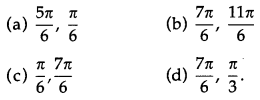Solution:
(b) $$\frac{7 \pi}{6}, \frac{11 \pi}{6}$$

Question 2.
The principal solution of equation cot θ = $$\sqrt {3}$$ ___________.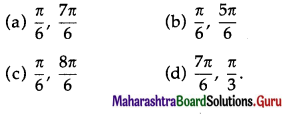Solution:
(a) $$\frac{\pi}{6}, \frac{7 \pi}{6}$$

Question 3.
The general solution of sec x = $$\sqrt {2}$$ is __________.
(a) 2nπ ± $$\frac{\pi}{4}$$, n ∈ Z
(b) 2nπ ± $$\frac{\pi}{2}$$, n ∈ Z
(c) nπ ± $$\frac{\pi}{2}$$, n ∈ Z
(d) 2nπ ± $$\frac{\pi}{3}$$, n ∈ Z
Solution:
(a) 2nπ ± $$\frac{\pi}{4}$$, n ∈ Z

Question 4.
If cos pθ = cosqθ, p ≠ q rhen ________.
(a) θ = $$\frac{2 n \pi}{p \pm q}$$
(b) θ = 2nπ
(c) θ = 2nπ ± p
(d) nπ ± q
Solution:
(a) θ = $$\frac{2 n \pi}{p \pm q}$$Question 5.
If polar co-ordinates of a point are $$\left(2, \frac{\pi}{4}\right)$$ then its cartesian co-ordinates are ______.
(a) (2, $$\sqrt {2}$$ )
(b) ($$\sqrt {2}$$, 2)
(c) (2, 2)
(d) ($$\sqrt {2}$$ , $$\sqrt {2}$$)
Solution:
(d) ($$\sqrt {2}$$ , $$\sqrt {2}$$)

Question 6.
If $$\sqrt {3}$$ cosx – sin x = 1, then general value of x is _________.
(a) 2nπ ± $$\frac{\pi}{3}$$
(b) 2nπ ± $$\frac{\pi}{6}$$
(c) 2nπ ± $$\frac{\pi}{3}-\frac{\pi}{6}$$
(d) nπ + (-1)n$$\frac{\pi}{3}$$
Solution:
(c) 2nπ ± $$\frac{\pi}{3}-\frac{\pi}{6}$$

Question 7.
In ∆ABC if ∠A = 45°, ∠B = 60° then the ratio of its sides are _________.
(a) 2 : $$\frac{\pi}{2}$$ : $$\frac{\pi}{3}$$ + 1
(b) $$\frac{\pi}{2}$$ : 2 : $$\frac{\pi}{3}$$ + 1
(c) 2 $$\frac{\pi}{2}$$ : $$\frac{\pi}{2}$$ : $$\frac{\pi}{3}$$
(d) 2 : 2 $$\frac{\pi}{2}$$ : $$\frac{\pi}{3}$$ + 1
Solution:
(a) 2 : $$\frac{\pi}{2}$$ : $$\frac{\pi}{3}$$ + 1Question 8.
In ∆ABC, if c2 + a2 – b2 = ac, then ∠B = __________.
(a) $$\frac{\pi}{4}$$
(b) $$\frac{\pi}{3}$$
(c) $$\frac{\pi}{2}$$
(d) $$\frac{\pi}{6}$$
Solution:
(b) $$\frac{\pi}{3}$$

Question 9.
In ABC, ac cos B – bc cos A = ____________.
(a) a2 – b2
(b) b2 – c2
(c) c2 – a2
(d) a2 – b2 – c2
Solution:
(a) a2 – b2

Question 10.
If in a triangle, the are in A.P. and b : c = $$\sqrt {3}$$ : $$\sqrt {2}$$ then A is equal to __________.
(a) 30°
(b) 60°
(c) 75°
(d) 45°
Solution:
(c) 75°Question 11.
cos-1$$\left(\cos \frac{7 \pi}{6}\right)$$ = ________.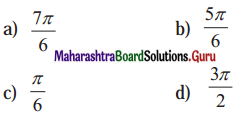Question 12.
The value of cot (tan-1 2x + cot-1 2x) is __________.
(a) 0
(b) 2x
(c) π + 2x
(d) π – 2x
Solution:
(a) 0

Question 13.
The principal value of sin-1$$\left(-\frac{\sqrt{3}}{2}\right)$$ is ____________.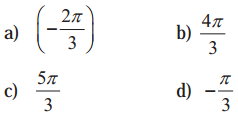Solution:
(d) $$-\frac{\pi}{3}$$Question 14.
If sin-1$$\frac{4}{5}$$ + cos-1$$\frac{,12}{13}$$ = sin-1 ∝, then ∝ = _____________.
(a) $$\frac{63}{65}$$
(b) $$\frac{62}{65}$$
(c) $$\frac{61}{65}$$
(d) $$\frac{60}{65}$$
Solution:
(a) $$\frac{63}{65}$$

Question 15.
If tan-1(2x) + tan-1(3x) = $$\frac{\pi}{4}$$, then x = ________.
(a) -1
(b) $$\frac{1}{6}$$
(c) $$\frac{2}{6}$$
(d) $$\frac{3}{2}$$
Solution:
(b) $$\frac{1}{6}$$

Question 16.
2 tan-1$$\frac{1}{3}$$ + tan-1$$\frac{1}{7}$$ = ______.
(a) tan-1$$\frac{4}{5}$$
(b) $$\frac{\pi}{2}$$
(c) 1
(d) $$\frac{\pi}{4}$$
Solution:
(d) $$\frac{\pi}{4}$$Question 17.
tan (2 tan-1$$\left(\frac{1}{5}\right)-\frac{\pi}{4}$$) = ______.
(a) $$\frac{17}{7}$$
(b) $$-\frac{17}{7}$$
(c) $$\frac{7}{17}$$
(d) $$-\frac{7}{17}$$
Solution:
(d) $$-\frac{7}{17}$$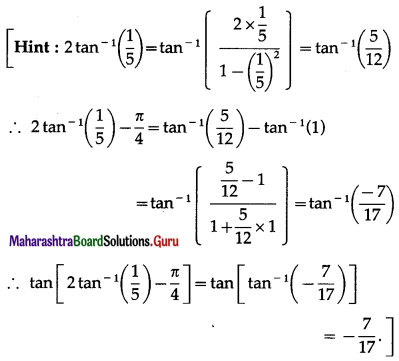Question 18.
The principal value branch of sec-1 x is __________.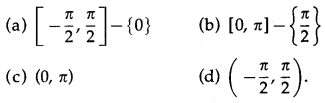Solution:
(b) [0, π] – {$$\frac{\pi}{2}$$}

Question 19.
cos[tan-1$$\frac{1}{3}$$ + tan-1$$\frac{1}{2}$$] = ________.
(a) $$\frac{1}{\sqrt{2}}$$
(b) $$\frac{\sqrt{3}}{2}$$
(c) $$\frac{1}{2}$$
(d) $$\frac{\pi}{4}$$
Solution:
(a) $$\frac{1}{\sqrt{2}}$$Question 20.
If tan θ + tan 2θ + tan 3θ = tan θ∙tan 2θ∙tan 3θ, then the general value of the θ is _______.
(a) nπ
(b) $$\frac{n \pi}{6}$$
(c) nπ ± $$\frac{n \pi}{4}$$
(d) $$\frac{n \pi}{2}$$
Solution:
(b) $$\frac{n \pi}{6}$$
[Hint: tan(A + B + C) = $$\frac{\tan A+\tan B+\tan C-\tan A \cdot \tan B \cdot \tan C}{1-\tan A \cdot \tan B-\tan B \cdot \tan C-\tan C \cdot \tan A}$$
Since , tan θ + tan 2θ + tan 3θ = tan θ ∙ tan 2θ ∙ tan 3θ,
we get, tan (θ + 2θ + 3θ) = θ
∴ tan6θ = 0
∴ 6θ = nπ, θ = $$\frac{n \pi}{6}$$.]

Question 21.
If any ∆ABC, if a cos B = b cos A, then the triangle is ________.
(a) Equilateral triangle
(b) Isosceles triangle
(c) Scalene
(d) Right angled
Solution:
(b) Isosceles triangle

II: Solve the following
Question 1.
Find the principal solutions of the following equations :
(i) sin2θ = $$-\frac{1}{2}$$
Solution:
sin2θ = $$-\frac{1}{2}$$
Since, θ ∈ (0, 2π), 2∈ ∈ (0, 4π)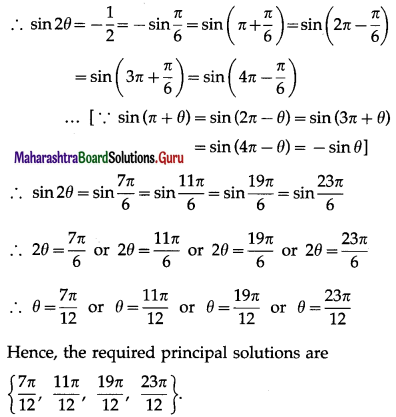(ii) tan3θ = -1
Solution:
Since, θ ∈ (0, 2π), 3∈ ∈ (0, 6π)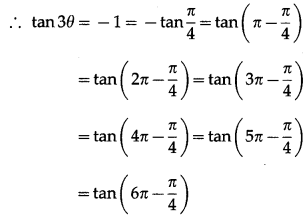… [∵ tan(π – θ) = tan(2π – θ) = tan(3π – θ)
= tan (4π – θ) = tan (5π – θ) = tan (6π – θ) = -tan θ]
∴ tan3θ = tan$$\frac{3 \pi}{4}$$ = tan$$\frac{7 \pi}{4}$$ = tan$$\frac{11 \pi}{4}$$ = tan$$\frac{15 \pi}{4}$$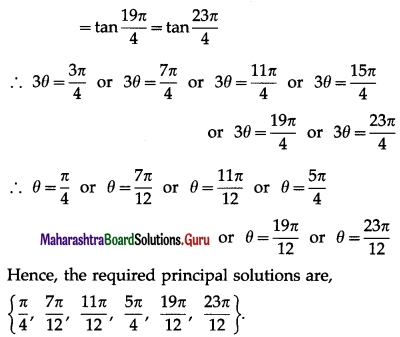(iii) cotθ = 0
Solution:
cotθ = 0
Since θ ∈ (0, 2π),
cotθ = 0 = cot $$\frac{\pi}{2}$$ = cot (π + $$\frac{\pi}{2}$$ …[∵ cos(π + θ) = cotθ]
∴ cotθ = cot$$\frac{\pi}{2}$$ = cot$$\frac{3 \pi}{2}$$
∴ θ = $$\frac{\pi}{2}$$ or θ = $$\frac{3 \pi}{2}$$
Hence, the required principal solutions are $$\left\{\frac{\pi}{2}, \frac{3 \pi}{2}\right\}$$Question 2.
Find the principal solutions of the following equations :
(i) sin2θ = $$-\frac{1}{\sqrt{2}}$$
Solution:

(ii) tan5θ = -1
Solution:

(iii) cot2θ = 0
Solution:

Question 3.
Which of the following equations have no solutions ?
(i) cos 2θ = $$\frac{1}{3}$$
Solution:
cos 2θ = $$\frac{1}{3}$$
Since $$\frac{1}{3}$$ ≤ cosθ ≤ 1 for any θ
cos2θ = $$\frac{1}{3}$$ has solution

(ii) cos2 θ = -1
Solution:
cos2θ = -1
This is not possible because cos2θ ≥ 0 for any θ.
∴ cos2θ = -1 does not have any solution.

(iii) 2 sinθ = 3
Solution:
2 sin θ = 3 ∴ sin θ = $$\frac{3}{2}$$
This is not possible because -1 ≤ sin θ ≤ 1 for any θ.
∴ 2 sin θ = 3 does not have any solution.

(iv) 3 sin θ = 5
Solution:
3 sin θ = 5
∴ sin θ = $$\frac{5}{3}$$
This is not possible because -1 ≤ sin θ ≤ 1 for any θ.
∴ 3 sin θ = 5 does not have any solution.

Question 4.
Find the general solutions of the following equations :
(i) tanθ = $$-\sqrt {x}$$
Solution:
The general solution of tan θ = tan ∝ is
θ = nπ + ∝, n ∈ Z.
Now, tanθ = $$-\sqrt {x}$$
∴ tanθ = tan$$\frac{\pi}{3}$$ …[∵ tan$$\frac{\pi}{3}$$ = $$\sqrt {3}$$]
∴ tanθ = tan$$\left(\pi-\frac{\pi}{3}\right)$$ …[∵ tan(π – θ) = -tanθ]
∴ tanθ = tan$$\frac{2 \pi}{3}$$
∴ the required general solution is
θ = nπ + $$\frac{2 \pi}{3}$$, n ∈ Z.

(ii) tan2θ = 3
Solution:
The general solution of tan2θ = tan2∝ is
θ = nπ ± ∝, n ∈ Z.
Now, tan2θ = 3 = ($$\sqrt {x}$$)2
∴ tan2θ = (tan$$\frac{\pi}{3}$$)2 …[∵ tan$$\frac{\pi}{3}$$ = $$\sqrt {3}$$]
∴ tan2θ = tan2$$\frac{\pi}{3}$$
∴ the required general solution is
θ = nπ ± $$\frac{\pi}{3}$$, n ∈ Z.

(iii) sin θ – cosθ = 1
Solution:
∴ cosθ – sin θ = -1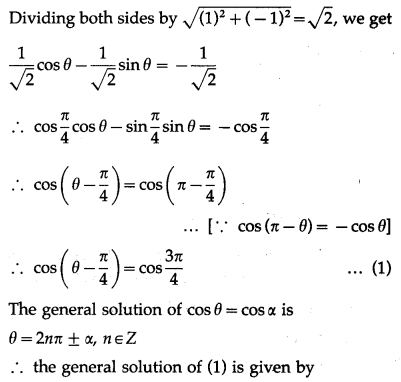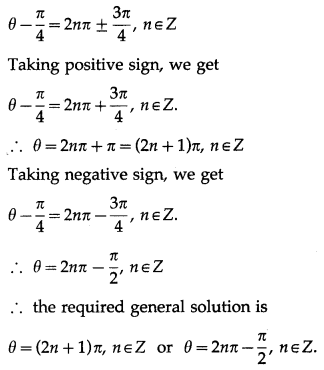(iv) sin2θ – cos2θ = 1
Solution:
sin2θ – cos2θ = 1
∴ cos2θ – sin2θ = -1
∴ cos2θ = cosπ …(1)
The general solution of cos θ = cos ∝ is
θ = 2nπ ± ∝, n ∈ Z
∴ the general solution of (1) is given by
2θ = 2nπ ± π, n ∈ Z
∴ θ = nπ ± $$\frac{\pi}{2}$$, n ∈ ZQuestion 5.
In ∆ABC prove that cos $$\left(\frac{A-B}{2}\right)=\left(\frac{a+b}{c}\right)$$ sin $$\frac{C}{2}$$
Solution:
By the sine rule,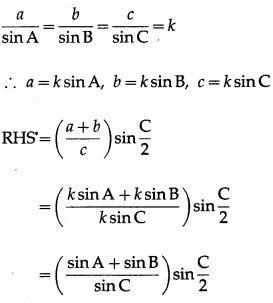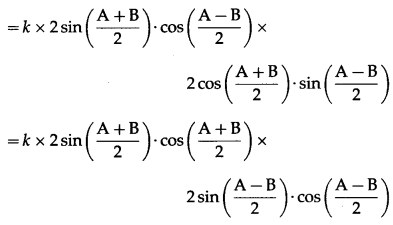Question 6.
With usual notations prove that $$\frac{\sin (A-B)}{\sin (A+B)}=\frac{a^{2}-b^{2}}{c^{2}}$$.
Solution:
By the sine rule,
$$\frac{a}{\sin \mathrm{A}}$$ = $$\frac{b}{\sin \mathrm{B}}$$ = $$\frac{c}{\sin \mathrm{C}}$$ = k
∴ a = ksinA, b = ksinB, c = ksinC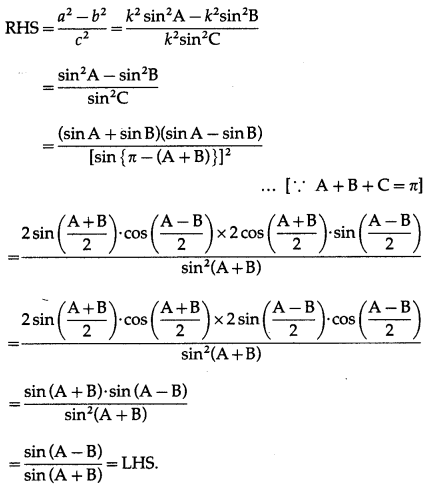Question 7.
In ∆ABC prove that (a – b)2 2cos2$$\frac{\mathrm{C}}{2}$$ + (a + b)2 sin2$$\frac{\mathrm{C}}{2}$$ = c2.
Solution:
LHS (a – b)2 2cos2$$\frac{\mathrm{C}}{2}$$ + (a + b)2 sin2$$\frac{\mathrm{C}}{2}$$
= (a2 + b2 – 2ab) cos2$$\frac{\mathrm{C}}{2}$$ + (a2 + b2 + 2ab) sin$$\frac{\mathrm{C}}{2}$$2
= (a2 + b2) cos2$$\frac{\mathrm{C}}{2}$$ – 2ab cos2$$\frac{\mathrm{C}}{2}$$ + (a2 + b2) sin2$$\frac{\mathrm{C}}{2}$$ + 2ab sin2$$\frac{\mathrm{C}}{2}$$
= (a2 + b2) (cos2$$\frac{\mathrm{C}}{2}$$ + sin2$$\frac{\mathrm{C}}{2}$$) – 2ab(cos2$$\frac{\mathrm{C}}{2}$$ – sin2$$\frac{\mathrm{C}}{2}$$)
= a2 + b2 – 2ab cos C
= c2 = RHS.Question 8.
In ∆ABC if cosA = sin B – cos C then show that it is a right angled triangle.
Solution:
cos A= sin B – cos C
∴ cos A + cos C = sin B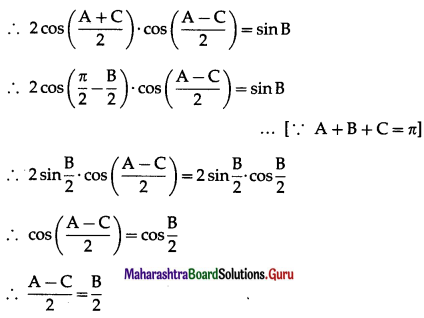∴ A – C = B
∴ A = B + C
∴ A + B + C = 180° gives
A + A = 180°
∴ 2A = 180 ∴ A = 90°
∴ ∆ ABC is a rightangled triangle.

Question 9.
If $$\frac{\sin A}{\sin C}=\frac{\sin (A-B)}{\sin (B-C)}$$ then show that a2, b2, c2, are in A.P.
Solution:
By sine rule,
$$\frac{\sin \mathrm{A}}{a}$$ = $$\frac{\sin \mathrm{B}}{b}$$ = $$\frac{\sin \mathrm{C}}{c}$$ = k
∴ sin A = ka, sin B = kb,sin C = kc
Now, $$\frac{\sin A}{\sin C}=\frac{\sin (A-B)}{\sin (B-C)}$$
∴ sinA∙sin(B – C) = sinC∙sin(A -B)
∴ sin [π – (B + C)] ∙ sin (B – C)
= sin [π – (A + B)]∙sin (A – B) … [∵ A + B + C = π]
∴ sin(B + C) ∙ sin(B – C) = sin (A + B) ∙ sin (A – B)
∴ sin2B – sin2C = sin2A – sin2B
∴ 2 sin2B = sin2A + sin2C
∴ 2k2b2 = k2a2 + k2c2
∴ 2b2 = a2 + c2
Hence, a2, b2, c2 are in A.P.

Question 10.
Solve the triangle in which a = ($$\sqrt {3}$$ + 1), b = ($$\sqrt {3}$$ – 1) and ∠C = 60°.
Solution:
Given : a = $$\sqrt {3}$$ + 1, b = $$\sqrt {3}$$ – 1 and ∠C = 60°.
By cosine rule,
c2 = a2 + b2 – 2ab cos C
= ($$\sqrt {3}$$ + 1)2 + ($$\sqrt {3}$$ – 1)2 – 2($$\sqrt {3}$$ + 1)($$\sqrt {3}$$ – 1)cos60°
= 3 + 1 + 2$$\sqrt {3}$$ + 3+ 1 – 2$$\sqrt {3}$$ – 2(3 – 1)$$\left(\frac{1}{2}\right)$$
= 8 – 2 = 6
∴ c = $$\sqrt {6}$$ …[∵ c > 0)
By sine rule,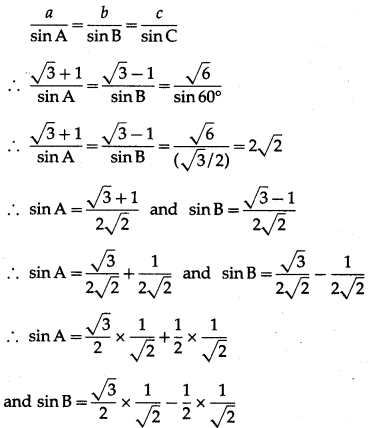∴ sin A = sin 60° cos 45° + cos 60° sin 45°
and sin B = sin 60° cos 45° – cos 60° sin 45°
∴ sin A = sin (60° + 45°) – sin 105°
and sin B = sin (60° – 45°) = sin 15°
∴ A = 105° and B = 15°
Hence, A = 105°, B 15° and C = $$\sqrt {6}$$ units.

Question 11.
In ∆ABC prove the following :
(i) a sin A – b sin B = c sin (A – B)
Solution:
By sine rule,
$$\frac{a}{\sin \mathrm{A}}$$ = $$\frac{b}{\sin \mathrm{B}}$$ = $$\frac{c}{\sin \mathrm{C}}$$ = k
∴ a = ksinA, b = ksinB, c = ksinC,
LHS = a sin A – b sinB
= ksinA∙sinA – ksinB∙sinB
= k (sin2A – sin2B)
= k (sin A + sin B)(sin A – sin B)= k × sin (A + B) × sin (A – B)
= ksin(π – C)∙sin(A – B) … [∵ A + B + C = π]
= k sinC∙sin (A – B)
= c sin (A – B) = RHS.

(ii) $$\frac{c-b \cos A}{b-c \cos A}=\frac{\cos B}{\cos C}$$.
Solution: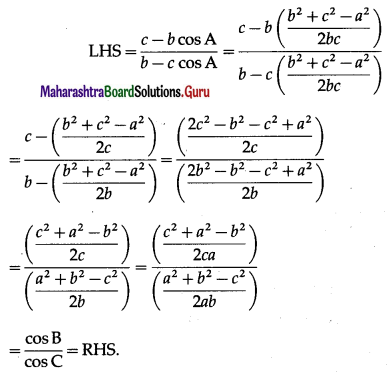(iii) a2 sin (B – C) = (b2 – c2) sinA
Solution:
By sine rule,
$$\frac{a}{\sin \mathrm{A}}$$ = $$\frac{b}{\sin \mathrm{B}}$$ = $$\frac{c}{\sin \mathrm{C}}$$ = k
∴ a = ksinA, b = ksinB, c = ksinC
RHS = (b2 – c2) sin A
= (k2sin2B – k2sin2C)sin A
= k2(sin2B – sin2C) sin A
= k2(sin B + sin C)(sin B – sin C) sin A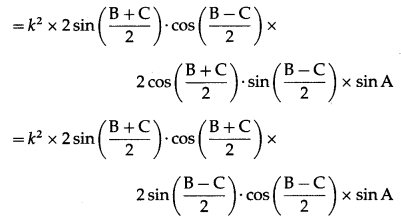= k2 × sin (B + C) × sin (B – C) × sin A
= k2∙sin(π – A)∙sin(B – C)∙sinA … [∵ A + B + C = π]
= k2sin A∙sin (B – C)∙sin A
= (k sin A)2∙sin (B – C)
= a2sin (B – C) = LHS.

(iv) ac cos B – bc cos A = (a2 – b2).
Solution:
LHS = ac cos B – bc cos A
= ac$$\left(\frac{c^{2}+a^{2}-b^{2}}{2 c a}\right)$$ – bc$$\left(\frac{b^{2}+c^{2}-a^{2}}{2 b c}\right)$$
=$$\frac{1}{2}$$(c2 + a2 – b2) – $$\frac{1}{2}$$(b2 + c2 – a2)
= $$\frac{1}{2}$$(c2 + a2 – b2 – b2 – c2 + a2)
= $$\frac{1}{2}$$(2a2 – 2b2) = a2 – b2 = RHS.(v) $$\frac{\cos A}{a}+\frac{\cos B}{b}+\frac{\cos C}{c}=\frac{a^{2}+b^{2}+c^{2}}{2 a b c}$$ .
Solution: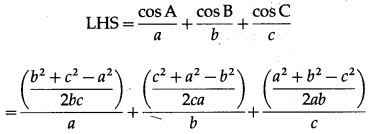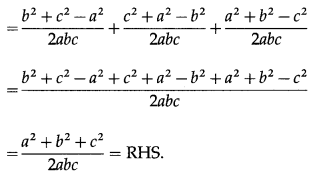(vi) $$\frac{\cos 2 \mathrm{~A}}{a^{2}}-\frac{\cos 2 \mathrm{~B}}{b^{2}}=\frac{1}{a^{2}}-\frac{1}{b^{2}}$$.
Solution:
By sine rule,
$$\frac{\sin \mathrm{A}}{a}=\frac{\sin \mathrm{B}}{b}$$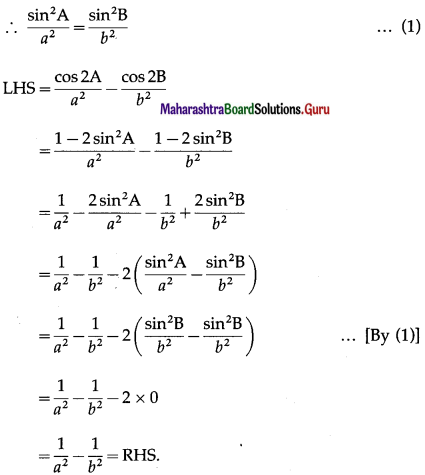(vii) $$\frac{b-c}{a}=\frac{\tan \frac{B}{2}-\tan \frac{C}{2}}{\tan \frac{B}{2}+\tan \frac{C}{2}}$$
Solution:
By sine rule,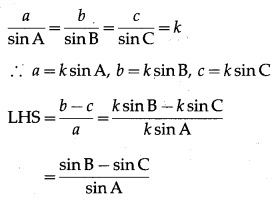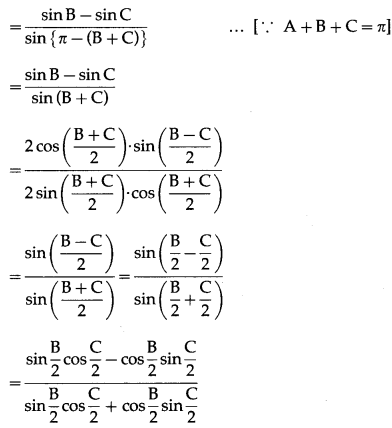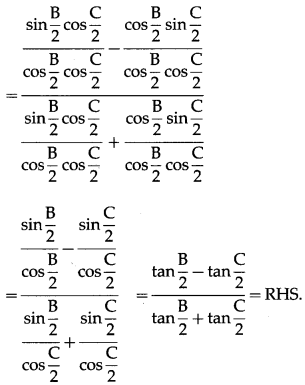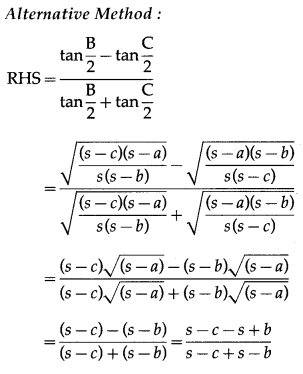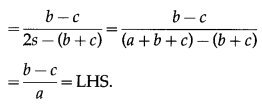Question 12.
In ∆ABC if a2, b2, c2, are in A.P. then cot$$\frac{A}{2}$$, cot$$\frac{B}{2}$$, cot$$\frac{C}{2}$$ are also in A.P.
Question is modified
In ∆ABC if a, b, c, are in A.P. then cot$$\frac{A}{2}$$, cot$$\frac{B}{2}$$, cot$$\frac{C}{2}$$ are also in A.P.
Solution:
a, b, c, are in A.P.
∴ 2b = a + c …(1)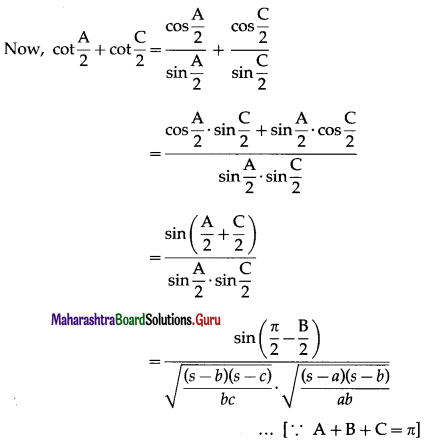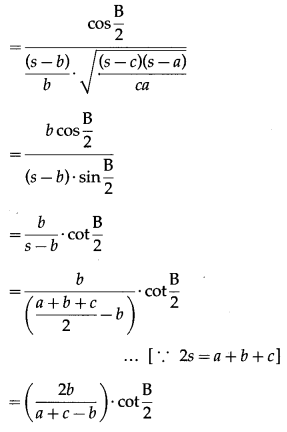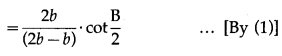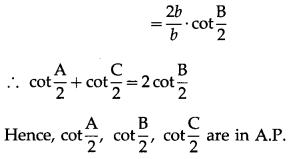Question 13.
In ∆ABC if ∠C = 90º then prove that sin(A – B) = $$\frac{a^{2}-b^{2}}{a^{2}+b^{2}}$$
Solution:
In ∆ABC, if ∠C = 90º
∴ c2 = a2 + b2 …(1)
By sine rule,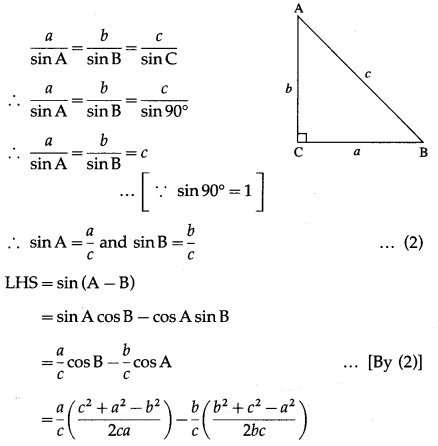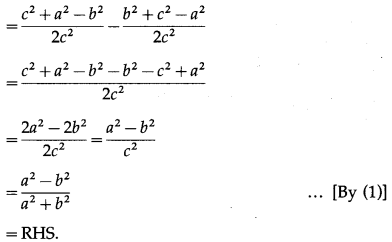Question 14.
In ∆ABC if $$\frac{\cos A}{a}=\frac{\cos B}{b}$$, then show that it is an isosceles triangle.
Solution:
Given : $$\frac{\cos A}{a}=\frac{\cos B}{b}$$ ….(1)
By sine rule,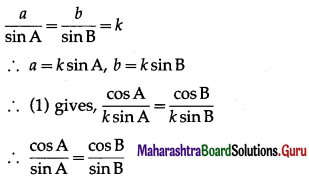∴ sin A cos B = cos A sinB
∴ sinA cosB – cosA sinB = 0
∴ sin (A – B) = 0 = sin0
∴ A – B = 0 ∴ A = B
∴ the triangle is an isosceles triangle.

Question 15.
In ∆ABC if sin2A + sin2B = sin2C then prove that the triangle is a right angled triangle.
Question is modified
In ∆ABC if sin2A + sin2B = sin2C then show that the triangle is a right angled triangle.
Solution:
By sine rule,
$$\frac{\sin \mathrm{A}}{a}$$ = $$\frac{\sin \mathrm{B}}{b}$$ = $$\frac{\sin \mathrm{C}}{c}$$ = k
∴ sin A = ka, sinB = kb, sin C = kc
∴ sin2A + sin2B = sin2C
∴ k2a2 + k2b2 = k2c2
∴ a2 + b2 = c2
∴ ∆ABC is a rightangled triangle, rightangled at C.Question 16.
In ∆ABC prove that a2(cos2B – cos2C) + b2(cos2C – cos2A) + c2(cos2A – cos2B) = 0.
Solution:
By sine rule,
$$\frac{a}{\sin \mathrm{A}}$$ = $$\frac{b}{\sin \mathrm{B}}$$ = $$\frac{c}{\sin \mathrm{C}}$$ = k
LHS = a2(cos2B – cos2C) + b2( cos2C – cos2A) + c2(cos2A – cos2B)
= k2sin2A [(1 – sin2B) – (1 – sin2C)] + k2sin2B [(1 – sin2C) – (1 – sin2A)] + k2sin2C[(1 – sin2A) – (1 – sin2B)]
= k2sin2A (sin2C – sin2B) + k2sin2B(sin2A – sin2C) + k2sin2C (sin2B – sin2A)
= k2(sin2A sin2C – sin2Asin2B + sin2A sin2B – sin2B sin2C + sin2B sin2C – sin2A sin2C)
= k2(0) = 0 = RHS.

Question 17.
With usual notations show that (c2 – a2 + b2) tan A = (a2 – b2 + c2) tan B = (b2 – c2 + a2) tan C.
Solution:
By sine rule,
$$\frac{a}{\sin A}$$ = $$\frac{b}{\sin B}$$ = $$\frac{c}{\sin C}$$ = k
∴ a = fksinA, b = ksinB, c = ksinC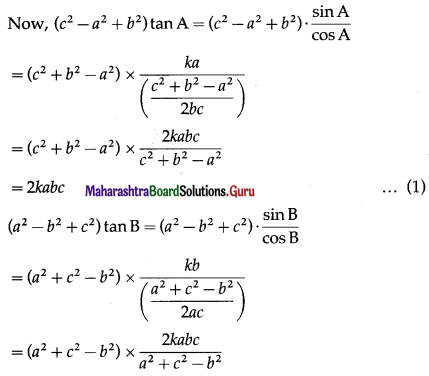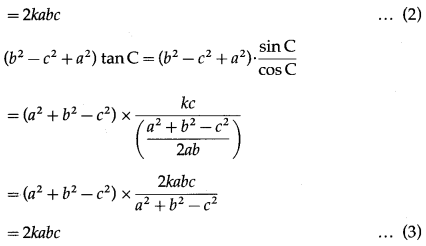From (1), (2) and (3), we get
(c2 – a2 + b2) tan A = (a2 – b2 + c2) tan B
= (b2 – c2 + a2) tan C.

Question 18.
In ∆ABC, if a cos2$$\frac{C}{2}$$ + c cos2$$\frac{A}{2}$$ = $$\frac{3 b}{2}$$, then prove that a , b ,c are in A.P.
Solution:
a cos2$$\frac{C}{2}$$ + c cos2$$\frac{A}{2}$$ = $$\frac{3 b}{2}$$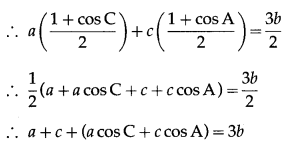∴ a + c + b = 3b …[∵ a cos C + c cos A = b]
∴ a + c = 2b
Hence, a, b, c are in A.P.Question 19.
Show that 2 sin-1$$\left(\frac{3}{5}\right)$$ = tan-1$$\left(\frac{24}{7}\right)$$.
Solution:
Let sin2$$\left(\frac{3}{5}\right)$$ = x.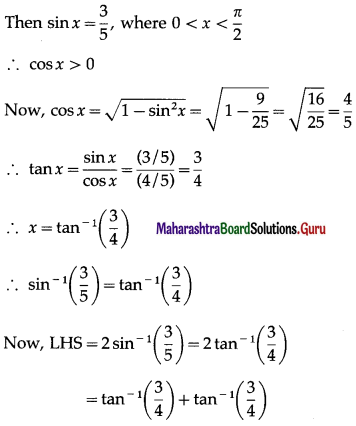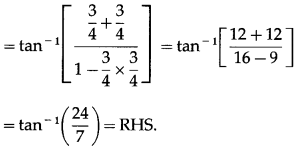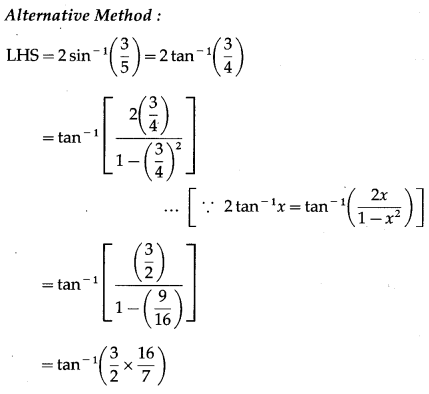∴ tan-1$$\left(\frac{24}{7}\right)$$ = RHS

Question 20.
Show that tan-1$$\left(\frac{1}{5}\right)$$ + tan-1$$\left(\frac{1}{7}\right)$$ + tan-1$$\left(\frac{1}{3}\right)$$ + tan-1$$\left(\frac{1}{8}\right)$$ = $$\frac{\pi}{4}$$.
Solution: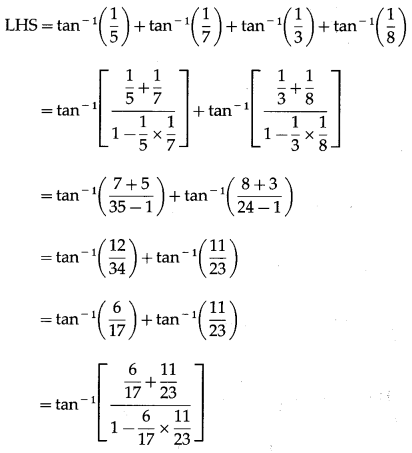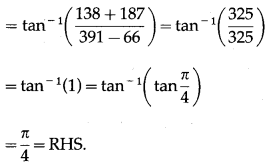Question 21.
Prove that tan-1$$\sqrt {x}$$ = $$\frac{1}{2}$$ cos-1$$\left(\frac{1-x}{1+x}\right)$$, if x ∈ [0, 1].
Solution:
Let tan-1$$\sqrt {x}$$ = y
∴ tan y = $$\sqrt {x}$$ ∴ x = tan2y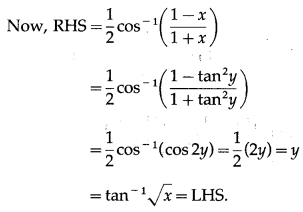Question 22.
Show that $$\frac{9 \pi}{8}-\frac{9}{4}$$ sin-1$$\frac{1}{3}$$ = $$\frac{9}{4}$$ sin-1$$\frac{2 \sqrt{2}}{3}$$.
Question is modified
Show that $$\frac{9 \pi}{8}-\frac{9}{4}$$ sin-1$$\left(\frac{1}{3}\right)$$ = $$\frac{9}{4}$$ sin-1$$\left(\frac{2 \sqrt{2}}{3}\right)$$.
Solution:
We have to show that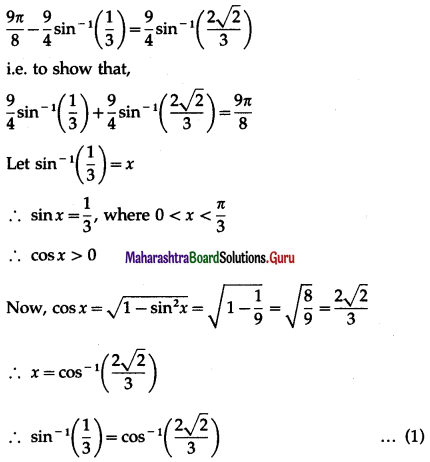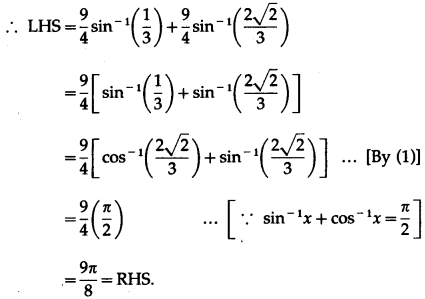Question 23.
Show that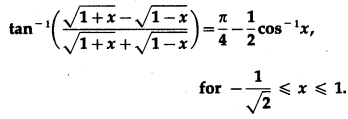Solution: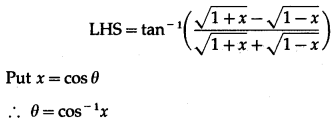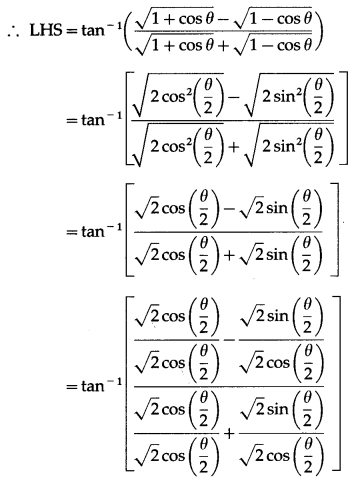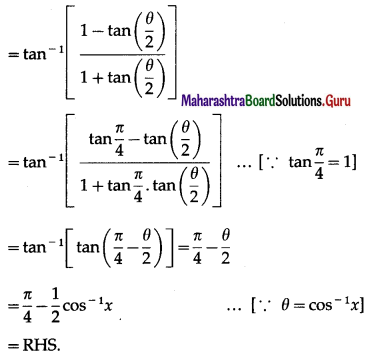Question 24.
If sin(sin-1$$\frac{1}{5}$$ + cos-1x) = 1, then find the value of x.
Solution:
sin(sin-1$$\frac{1}{5}$$ = 1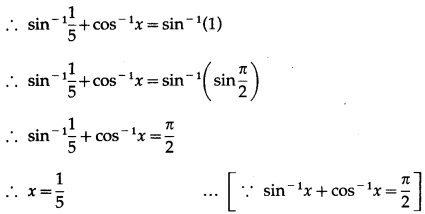Question 25.
If tan-1$$\left(\frac{x-1}{x-2}\right)$$ + tan-1$$\left(\frac{x+1}{x+2}\right)$$ = $$\frac{\pi}{4}$$ then find the value of x.
Solution:
tan-1$$\left(\frac{x-1}{x-2}\right)$$ + tan-1$$\left(\frac{x+1}{x+2}\right)$$ = $$\frac{\pi}{4}$$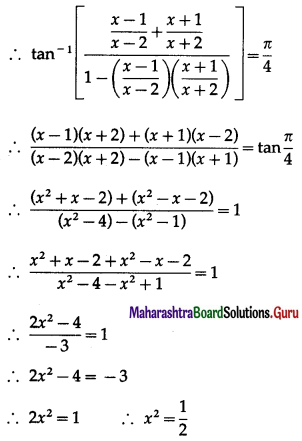∴ x = ±$$\frac{1}{\sqrt{2}}$$.

Question 26.
If 2 tan-1(cos x ) = tan-1(cosec x) then find the value of x.
Solution:
2 tan-1(cos x ) = tan-1(cosec x)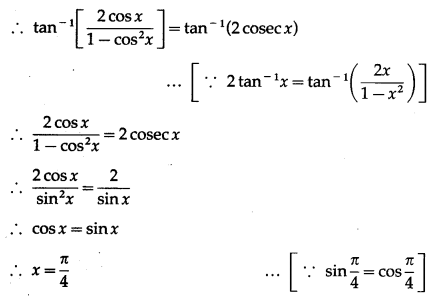Question 27.
Solve: tan-1$$\left(\frac{1-x}{1+x}\right)$$ = $$\frac{1}{2}$$(tan-1x), for x > 0.
Solution:
tan-1$$\left(\frac{1-x}{1+x}\right)$$ = $$\frac{1}{2}$$(tan-1x)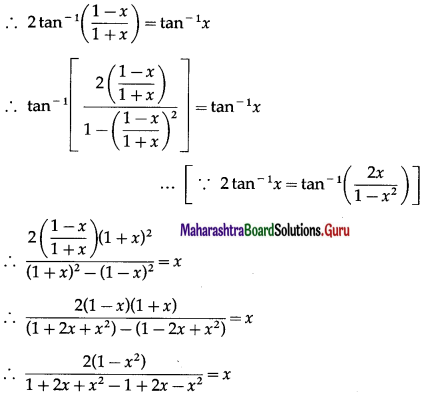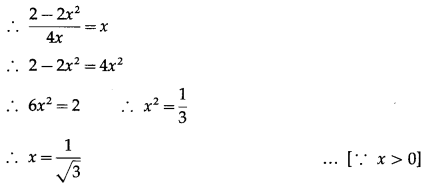Question 28.
If sin-1(1 – x) – 2sin-1x = $$\frac{\pi}{2}$$, then find the value of x.
Solution:
sin-1(1 – x) – 2sin-1x = $$\frac{\pi}{2}$$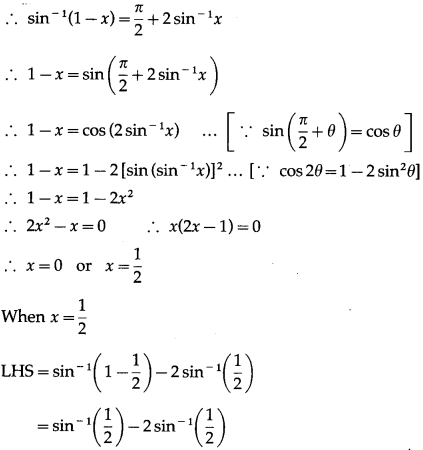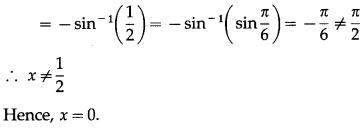Question 29.
If tan-12x + tan-13x = $$\frac{\pi}{4}$$, then find the value of x.
Question is modified
If tan-12x + tan-13x = $$\frac{\pi}{2}$$, then find the value of x.
Solution:
tan-12x + tan-13x = $$\frac{\pi}{4}$$
∴ tan-1$$\left(\frac{2 x+3 x}{1-2 x \times 3 x}\right)$$ = tan$$\frac{\pi}{4}$$, where 2x > 0, 3x > 0
∴ $$\frac{5 x}{1-6 x^{2}}$$ = tan$$\frac{\pi}{4}$$ = 1
∴ 5x = 1 – 6x2
∴ 6x2 + 5x – 1 = 0
∴ 6x2 + 6x – x – 1 = 0
∴ 6x(x +1) – 1(x + 1) = 0
∴ (x + 1)(6x – 1) = 0
∴ x = -1 or x = $$\frac{1}{6}$$
But x > 0 ∴ x ≠ -1
Hence, x = $$\frac{1}{6}$$

Question 30.
Show that tan-1$$\frac{1}{2}$$ – tan-1$$\frac{1}{4}$$ = tan-1$$\frac{2}{9}$$.
Solution:
LHS = tan-1$$\frac{1}{2}$$ – tan-1$$\frac{1}{4}$$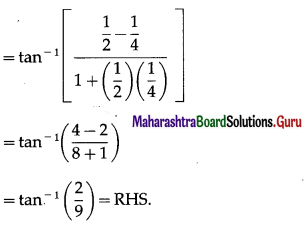Question 31.
Show that cot-1$$\frac{1}{3}$$ – tan-1$$\frac{1}{3}$$ = cot-1$$\frac{3}{4}$$.
Solution:
LHS = cot-1$$\frac{1}{3}$$ – tan-1$$\frac{1}{3}$$Question 32.
Show that tan-1$$\frac{1}{2}$$ = $$\frac{1}{3}$$ tan-1$$\frac{11}{2}$$.
Solution:
We have to show that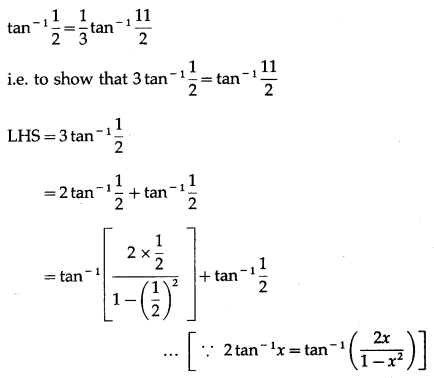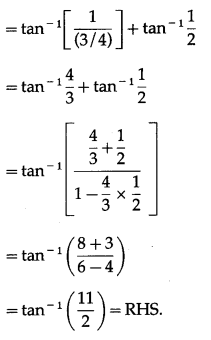Question 33.
Show that cos-1$$\frac{\sqrt{3}}{2}$$ + 2sin-1$$\frac{\sqrt{3}}{2}$$ = $$\frac{5 \pi}{6}$$
Solution: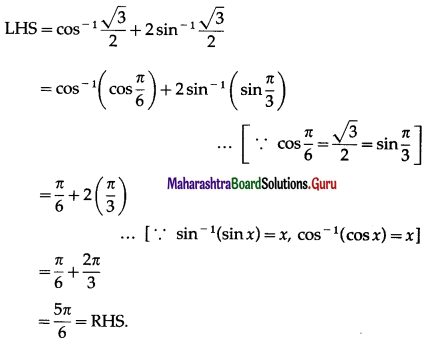Question 34.
Show that 2cot-1$$\frac{3}{2}$$ + sec-1$$\frac{13}{12}$$ = $$\frac{\pi}{2}$$
Solution: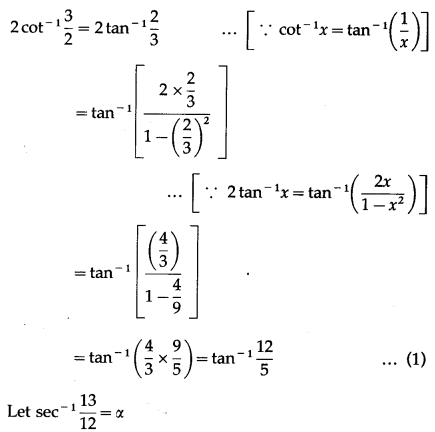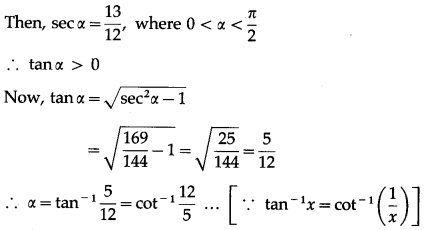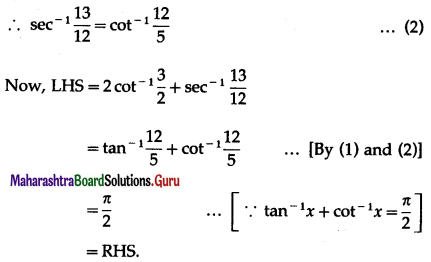Question 35.
Prove the following :
(i) cos-1 x = tan-1$$\frac{\sqrt{1-x^{2}}}{x}$$, if x < 0.
Question is modified
cos-1 x = tan-1$$\left(\frac{\sqrt{1-x^{2}}}{x}\right)$$, if x > 0.
Solution: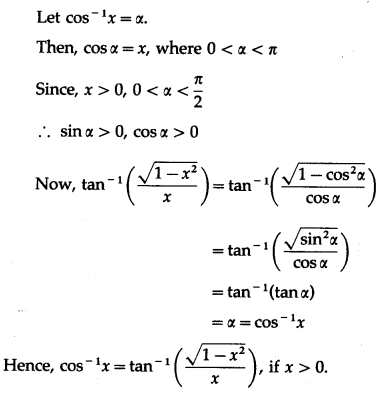(ii) cos-1 x = π + tan-1$$\frac{\sqrt{1-x^{2}}}{x}$$, if x < 0.
Solution: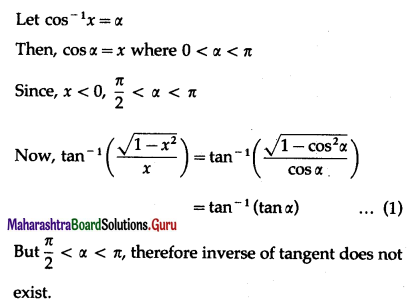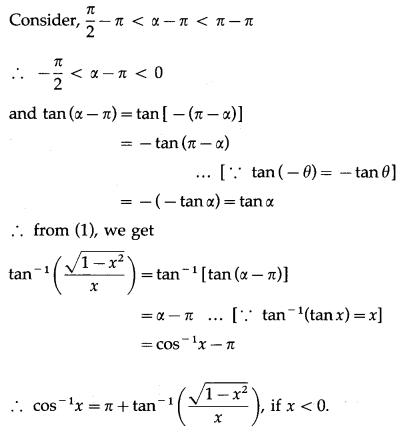Question 36.
If |x| < 1 , then prove that 2tan-1 x = tan-1$$\frac{2 x}{1-x^{2}}$$ = sin-1$$\frac{2 x}{1+x^{2}}$$ = cos-1$$\frac{1-x^{2}}{1+x^{2}}$$
Question is modified
If |x| < 1 , then prove that 2tan-1 x = tan-1$$\left(\frac{2 x}{1-x^{2}}\right)$$ = sin-1$$\left(\frac{2 x}{1+x^{2}}\right)$$ = cos-1$$\left(\frac{1-x^{2}}{1+x^{2}}\right)$$
Solution:
Let tan-1x = y
Then, x = tany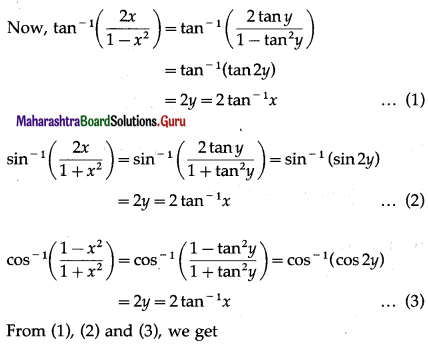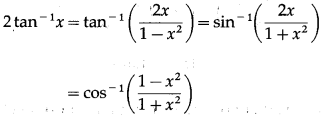Question 37.
If x, y, z, are positive then prove that tan-1$$\frac{x-y}{1+x y}$$ + tan-1$$\frac{y-z}{1+y z}$$ + tan-1$$\frac{z-x}{1+z x}$$ = 0
Solution: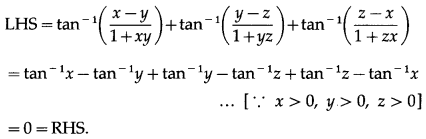Question 38.
If tan-1 x + tan-1 y + tan-1 z = $$\frac{\pi}{2}$$ then, show that xy + yz + zx = 1
Solution:
tan-1 x + tan-1 y + tan-1 z = $$\frac{\pi}{2}$$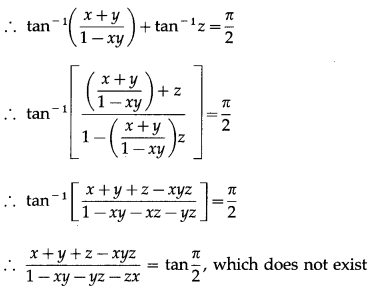∴ 1 – xy – yz – zx = 0
∴ xy + yz + zx = 1.Question 39.
If cos-1 x + cos-1 y + cos-1 z = π then show that x2 + y2 + z2 + 2xyz = 1.
Solution:
0 ≤ cos-1x ≤ π and
cos-1x + cos-1y+ cos-1z = 3π
∴ cos-1x = π, cos-1y = π and cos-1z = π
∴ x = y = z = cosπ = -1
∴ x2 + y2 + z2 + 2xyz
= (-1)2 + (-1)2 + (-1)2 + 2(-1)(-1)(-1)
= 1 + 1 + 1 – 2
= 3 – 2 = 1.